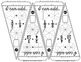Subject
Resource Type
File Type

PDF

(3 MB|8 pages)
Standards
Also included in:
1. This bundle includes 6 sets of fractions pennants for comparing, adding, subtracting, multiplying, dividing and converting. Each set includes math pennants for students to color, an optional student answer sheet and answer keys.Math pennants are a fun way to show off student work. Students love seei
\$23.00
\$12.50
Save \$10.50
• Product Description
• Standards

Students add fractions in this activity that doubles as classroom decor. Students color partitioned circles to show the given fractions, add the fractions together and then show the sum as a picture and as a fraction. All problems have unlike denominators that are related so that only one denominator needs to be changed in order for the two fractions to have a common denominator. Once a pennant is complete, it can be hung along a string in your classroom to show the world that, "Hey, we know how to add fractions!"

**Holiday version of this pennant is included in Fraction Ornaments mini-bundle.

Fractions range from halves to sixteenths.

Included are 20 pennants with fraction addition problems, an optional student answer sheet and an answer key. Each group of students can be given a set of pennants, crayons, scissors and either glue, tape or a stapler. Students can be in charge of cutting out the pennants. Groups can compete to see which group can complete the most pennants or your entire class can add pennants to one string as they complete each one.

You may also like:

Fractions Pennant Bundle

Add and subtract fractions with unlike denominators (including mixed numbers) by replacing given fractions with equivalent fractions in such a way as to produce an equivalent sum or difference of fractions with like denominators. For example, 2/3 + 5/4 = 8/12 + 15/12 = 23/12. (In general, 𝘢/𝘣 + 𝘤/𝘥 = (𝘢𝘥 + 𝘣𝘤)/𝘣𝘥.)
Total Pages
8 pages
Included
Teaching Duration
N/A
Report this Resource to TpT
Reported resources will be reviewed by our team. Report this resource to let us know if this resource violates TpT’s content guidelines.